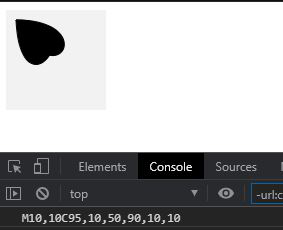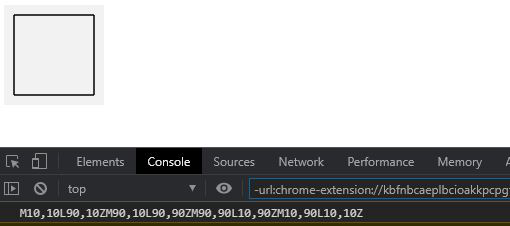Related Articles

# D3.js path.toString() Function

• Last Updated : 07 Aug, 2020

The path.toString() function in d3.js is used to return the string representation of the path of the shape drawn. The path representation is according to the SVG’s path data specification.

Syntax:

`path.toString() `

Parameters: This function does not accept any parameters.

Return Value: This function returns a string.

Example 1:

## HTML

 ```<``html` `lang``=``"en"``>`` ` `<``head``>``    ``<``meta` `charset``=``"UTF-8"``>``    ``<``meta` `name``=``"viewport"` `path1tent``=``        ``"width=device-width,initial-scale=1.0"``>`` ` `    ``<``script` `src``=``        ``"https://d3js.org/d3.v4.min.js"``>``    ```` ` `    ``<``style``>``        ``h1 {``            ``color: green;``        ``}`` ` `        ``svg {``            ``background-color: #f2f2f2;``        ``}`` ` `        ``.path2 {``            ``stroke: #000;``        ``}``    `````` ` `<``body``>``    ``<``div``>``        ``<``svg` `width``=``"100"` `height``=``"100"``>``            ``<``path` `class``=``"path2"``>``        ````    ```` ` `    ``<``script``>`` ` `        ``// Creating a path ``        ``var path = d3.path();``        ``path.moveTo(10, 10);``        ``path.bezierCurveTo(95, 10, 50, 90, 10, 10)``         ` `        ``// Printing the string of the path``        ``console.log(path.toString())``        ``path.bezierCurveTo(90, 10, 15, 110, 10, 10)``         ` `        ``// Closing the path ``        ``path.closePath();``        ``d3.select(".path2").attr("d", path); ``    `````` ` ``

Output: Path string for this shape is given in console.Example 2:

## HTML

 ```<``html` `lang``=``"en"``>`` ` `<``head``>``    ``<``meta` `charset``=``"UTF-8"``>``    ``<``meta` `name``=``"viewport"` `path1tent``=``        ``"width=device-width, initial-scale=1.0"``>`` ` `    ``<``script` `src``=``        ``"https://d3js.org/d3.v4.min.js"``>``    ```` ` `    ``<``style``>``        ``h1 {``            ``color: green;``        ``}`` ` `        ``svg {``            ``background-color: #f2f2f2;``        ``}`` ` `        ``.path2 {``            ``stroke: #000;``        ``}``    `````` ` `<``body``>``    ``<``div``>``        ``<``svg` `width``=``"100"` `height``=``"100"``>``            ``<``path` `class``=``"path2"``>``        ````    ```` ` `    ``<``script``>`` ` `        ``// Creating a path ``        ``var path = d3.path();``        ``path.moveTo(10, 10);``         ` `        ``// Making line to x:90 and y:10 ``        ``path.lineTo(90, 10);``         ` `        ``// Closing the path ``        ``path.closePath();``        ``path.moveTo(90, 10);``        ``path.lineTo(90, 90);``         ` `        ``// Closing the path ``        ``path.closePath();``        ``path.moveTo(90, 90);``        ``path.lineTo(10, 90);``         ` `        ``// Closing the path ``        ``path.closePath();``        ``path.moveTo(10, 90);``        ``path.lineTo(10, 10);``         ` `        ``// Closing the path ``        ``path.closePath();``         ` `        ``// Printing the string of the path``        ``console.log(path.toString())``        ``d3.select(".path2").attr("d", path); ``    `````` ` ``

Output: The path string for the square shape is given in the console.Hey geek! The constant emerging technologies in the world of web development always keeps the excitement for this subject through the roof. But before you tackle the big projects, we suggest you start by learning the basics. Kickstart your web development journey by learning JS concepts with our JavaScript Course. Now at it’s lowest price ever!

My Personal Notes arrow_drop_up